# ClarkVision.comHome Galleries Articles Reviews Best Gear Science New About Contact

## The Canon 5D Mark II Digital Camera Review: Sensor Noise, Dynamic Range, and Full Well Analysis

by Roger N. Clark

If you find the information on this site useful, please support Clarkvision and make a donation (link below).This page shows an analysis of noise, dynamic range, and full well capacity of a Canon 5D Mark II camera.

Procedures for performing this analysis are described in: Procedures for Evaluating Digital Camera Noise, Dynamic Range, and Full Well Capacities; Canon 1D Mark II Analysis

The lowest possible noise from a system detecting light is the noise due to Poisson statistics from the random rate of the arrival of photons. This is called photon statistics, or photon noise. Noise from the electronics will add to the photon noise. Noise in Canon 5D Mark II images is limited by photon statistics at high signal levels and by electronic noise from reading the sensor (called readout noise) and noise from the downstream electronics at very low signal levels. In the case of high signal levels, a system that is photon statistics limited enables us to directly measure how many photons the sensor captures, and by increasing the exposure, we can determine how many photons are required to saturate the sensor. That is called the full well capacity, or simply, maximum signal capacity. With data on the lowest noise to the highest signal, we can then determine the dynamic range of the sensor.

The data and analysis results below show how the canon 5D Mark II sensor performs. Table 1 shows the results and these results are shown on the graphs at Digital Sensor Performance summary for comparison with other cameras.

```              Table 1
-------------------------------------------------
Apparent  Maximum     Measured
ISO  Gain   Read Noise  signal    Dynamic range
e/DN  (electrons) (electrons)   stops

50  4.2     24.2       65700       11.41
100  4.1     23.5       59400       11.30
200  2.03    11.9       29700       11.20
400  1.01     6.4       14800       11.18
800  0.51     3.7        7425       10.97
1600  0.25     2.5        3710       10.54
3200  0.127    2.5        1860        9.54
6400  0.063    2.5         930        8.54
12800  0.032    2.5         460        7.54
25600  0.016                230

Pixel pitch: 6.4 microns.
S/N on 18% gray card, ISO 100 = 103.
Sensor Full Well Capacity at lowest ISO: 65,700 electrons.
Sensor dynamic range = 65700/2.5 = 26,280 = 14.7 stops.
ISO at unity gain (scaled to 12 bit) = 1600 (14-bit unity gain = ISO 404).
Low Light sensitivity Factor: 640.
Apparent Image Quality, AIQ = 109
All data derived by R. Clark, December, 2008.
```

Values in the above table are described at Digital Sensor Performance summary.

Table 2 shows the noise as a funnction of ISO in image form. The images illustrate several things: 1) lower banding noise at higher ISOs. 2) Better detection of smaller signals at higher ISOs (the random noise decreases). 3) At a certainl high ISO, improvements decrease, meaning there is no benefit to higher ISO. Note, ISO is a post sensor gain and does not increase sensitivity. Increasing ISO digitizes a smaller range (see Table 1) but does improve the noise floor up to a point. Some will find the pattern noise objectionable in ISO 1600 images in shadow areas and night photography. At lower ISOs, the pattern nosie is particularly bad. There is still significant pattern noise at the highest ISOs. For night and low light photography, I usually use ISO 3200 with this camera.

 Table 2a. Apparent Read Noise, Central Image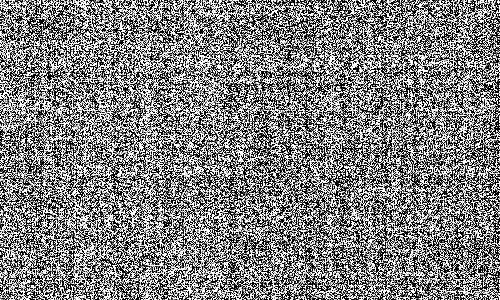ISO 50Image Range:-20.00 to 20.00 electrons about the meanCentral 500 x 300 pixel statistics:min= 6972 electronsmax= 7385 electronsmean= 7170 electronsstandard deviation= 44.54 electrons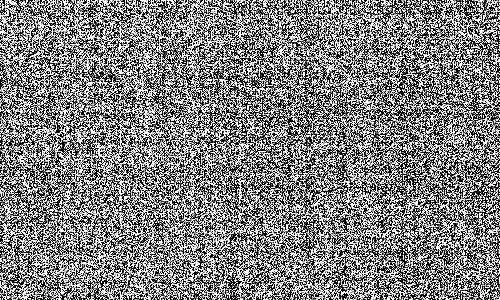ISO 100Image Range:-20.00 to 20.00 electrons about the meanCentral 500 x 300 pixel statistics:min= 6799 electronsmax= 7209 electronsmean= 7006 electronsstandard deviation= 43.33 electrons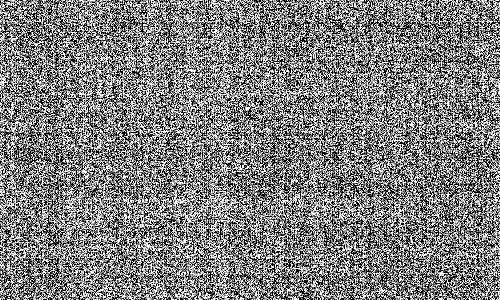ISO 200Image Range:-20.00 to 20.00 electrons about the meanCentral 500 x 300 pixel statistics:min= 3396 electronsmax= 3612 electronsmean= 3505 electronsstandard deviation= 21.91 electrons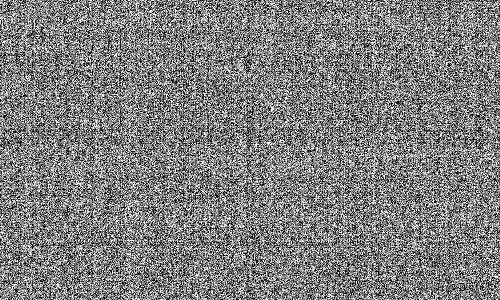ISO 400Image Range:-20.00 to 20.00 electrons about the meanCentral 500 x 300 pixel statistics:min= 1669 electronsmax= 1826 electronsmean= 1752 electronsstandard deviation= 11.57 electrons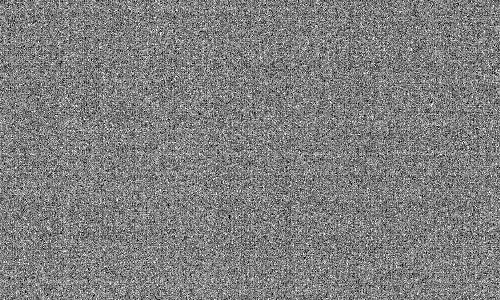ISO 800Image Range:-20.00 to 20.00 electrons about the meanCentral 500 x 300 pixel statistics:min= 817 electronsmax= 935 electronsmean= 876 electronsstandard deviation= 6.66 electrons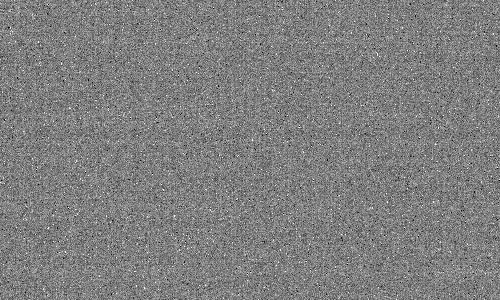ISO 1600Image Range:-20.00 to 20.00 electrons about the meanCentral 500 x 300 pixel statistics:min= 383 electronsmax= 489 electronsmean= 439 electronsstandard deviation= 4.47 electrons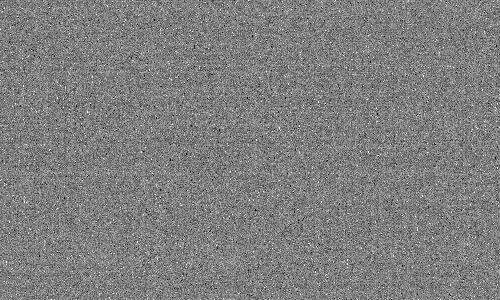ISO 3200Image Range:-20.00 to 20.00 electrons about the meanCentral 500 x 300 pixel statistics:min= 158 electronsmax= 277 electronsmean= 220 electronsstandard deviation= 4.49 electrons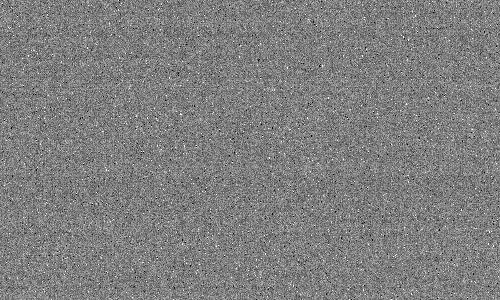ISO 6400Image Range:-20.00 to 20.00 electrons about the meanCentral 500 x 300 pixel statistics:min= 48 electronsmax= 167 electronsmean= 110 electronsstandard deviation= 4.49 electrons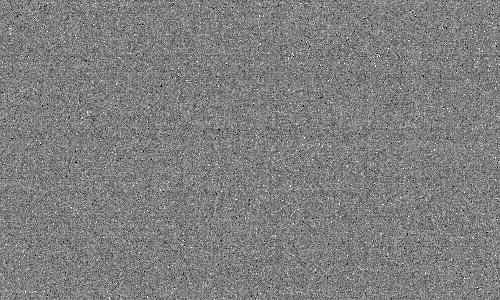ISO 12800Image Range:-20.00 to 20.00 electrons about the meanCentral 500 x 300 pixel statistics:min= 9 electronsmax= 120 electronsmean= 55 electronsstandard deviation= 4.42 electrons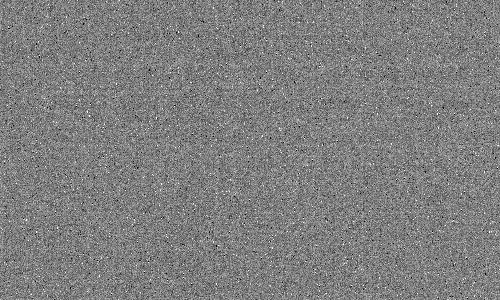ISO 25600Image Range:-20.00 to 20.00 electrons about the meanCentral 500 x 300 pixel statistics:min= 0 electronsmax= 91 electronsmean= 28 electronsstandard deviation= 4.47 electrons

 Table 2b. Apparent Read Noise, Full Image, sub-sampled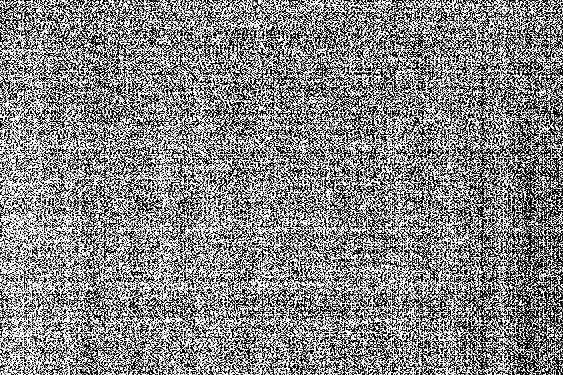ISO 50Image Range:-20.00 to 20.00 electrons about the meanFull image statistics:min= 6881 electronsmax= 10892 electronsmean= 7167 electronsstandard deviation= 45.79 electrons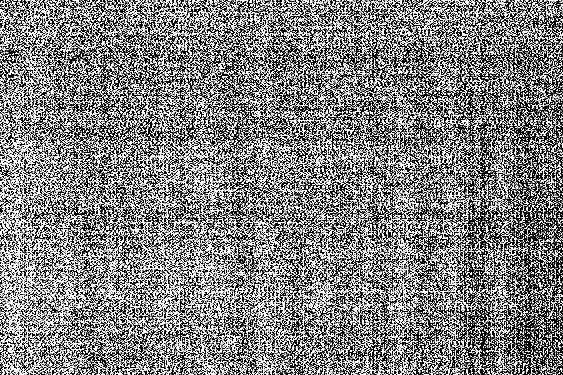ISO 100Image Range:-20.00 to 20.00 electrons about the meanFull image statistics:min= 6751 electronsmax= 10636 electronsmean= 7003 electronsstandard deviation= 44.88 electrons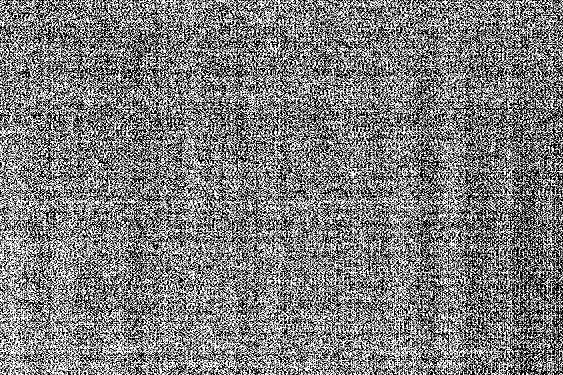ISO 200Image Range:-20.00 to 20.00 electrons about the meanFull image statistics:min= 3369 electronsmax= 6939 electronsmean= 3504 electronsstandard deviation= 22.54 electrons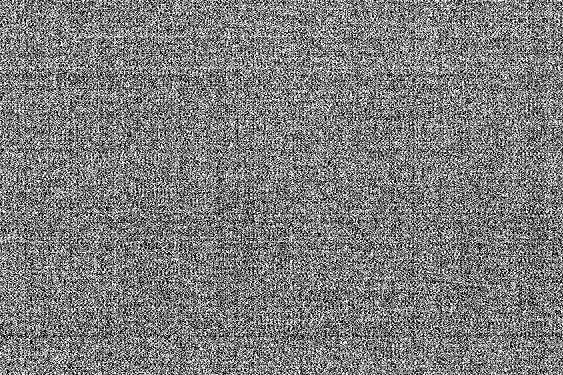ISO 400Image Range:-20.00 to 20.00 electrons about the meanFull image statistics:min= 1657 electronsmax= 5356 electronsmean= 1752 electronsstandard deviation= 11.62 electrons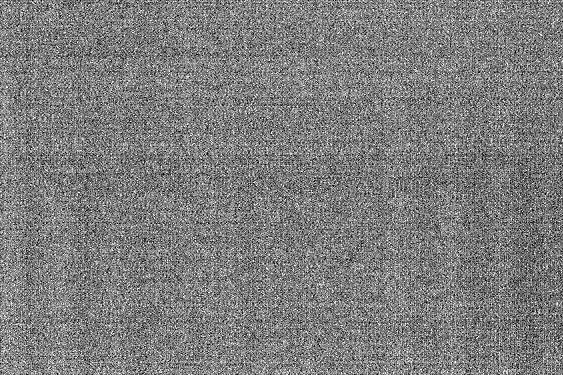ISO 800Image Range:-20.00 to 20.00 electrons about the meanFull image statistics:min= 792 electronsmax= 4593 electronsmean= 876 electronsstandard deviation= 6.88 electronsISO 1600Image Range:-20.00 to 20.00 electrons about the meanFull image statistics:min= 351 electronsmax= 4009 electronsmean= 439 electronsstandard deviation= 4.66 electrons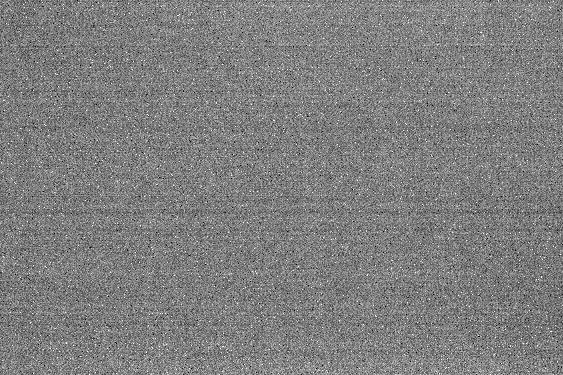ISO 3200Image Range:-20.00 to 20.00 electrons about the meanFull image statistics:min= 153 electronsmax= 3374 electronsmean= 220 electronsstandard deviation= 4.60 electrons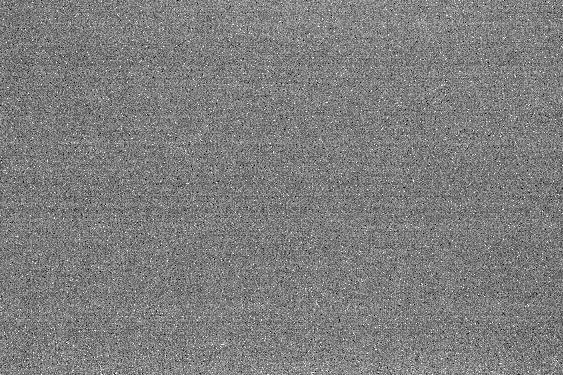ISO 6400Image Range:-20.00 to 20.00 electrons about the meanFull image statistics:min= 41 electronsmax= 1753 electronsmean= 111 electronsstandard deviation= 4.56 electrons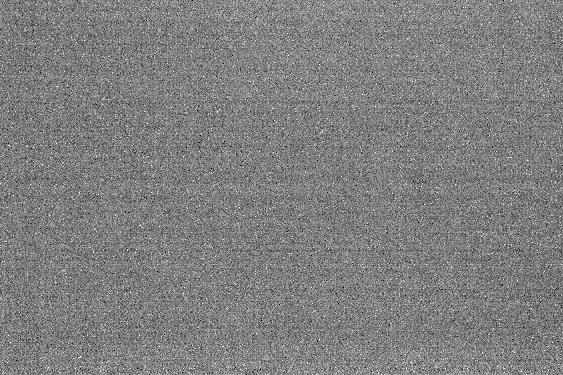ISO 12800Image Range:-20.00 to 20.00 electrons about the meanFull image statistics:min= 0 electronsmax= 868 electronsmean= 55 electronsstandard deviation= 4.50 electrons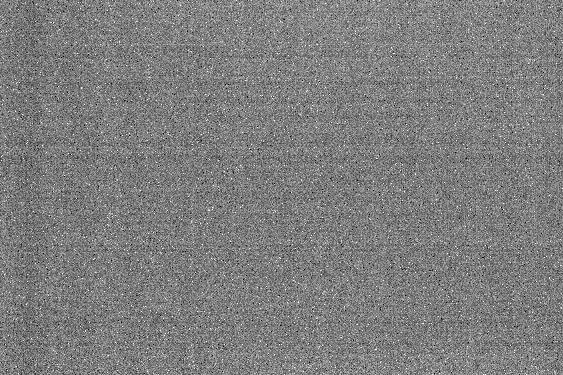ISO 25600Image Range:-20.00 to 20.00 electrons about the meanFull image statistics:min= 0 electronsmax= 442 electronsmean= 28 electronsstandard deviation= 4.58 electrons

If you find the information on this site useful, please support Clarkvision and make a donation (link below).References

Notes:

DN is "Data Number." That is the number in the file for each pixel. I'm quoting the luminance level (although red, green and blue are almost the same in the cases I cited).

16-bit signed integer: -32768 to +32767

16-bit unsigned integer: 0 to 65535

Photoshop uses signed integers, but the 16-bit tiff is unsigned integer (correctly read by ImagesPlus).

The sensor analysis was done with custom, in-house written software. Raw data were extracted from the camera raw files using DCRAW. Custom software read that data and all processing was done in 32-bit floating point.

 Home Galleries Articles Reviews Best Gear Science New About Contact

http://www.clarkvision.com/reviews/evaluation-canon-1dii

First published December 2010.
Last updated November 17, 2013.